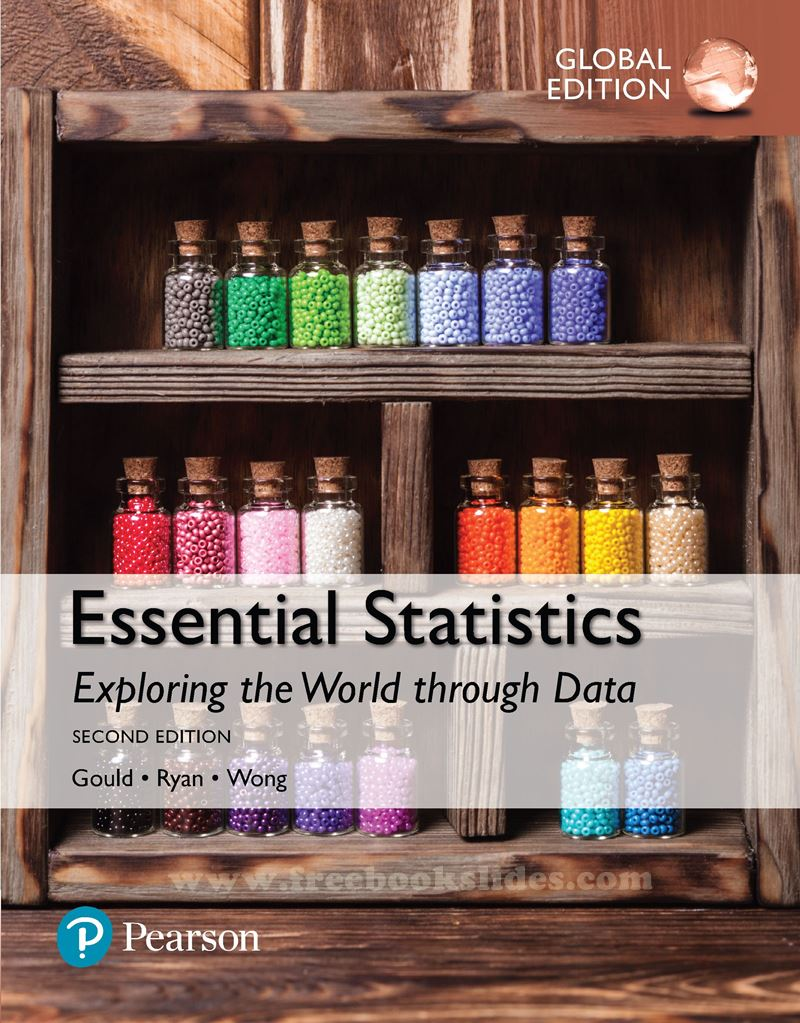### Ebook Essential Statistics 2e by Gould, Ryan, Wong (Global Edition)Essential Statistics : Exploring the World through Data
2nd Edition (Global Edition)
Author(s) : Robert Gould - Colleen N. Ryan - Rebecca Wong
Publisher : Pe*rson Education
PDF 21.67 MB

Table of Content:
1. Introduction to Data
1.1 What Are Data?
1.2 Classifying and Storing Data
1.3 Organizing Categorical Data
1.4 Collecting Data to Understand Causality
Exploring Statistics–Collecting a Table of Different Kinds of Data
2. Picturing Variation with Graphs
Case Study–Student-to-Teacher Ratio at Colleges
2.1 Visualizing Variation in Numerical Data
2.2 Summarizing Important Features of a Numerical Distribution
2.3 Visualizing Variation in Categorical Variables
2.4 Summarizing Categorical Distributions
2.5 Interpreting Graphs
Exploring Statistics–Personal Distance
3. Numerical Summaries of Center and Variation
Case Study–Living in a Risky World
3.1 Summaries for Symmetric Distributions
3.2 What’s Unusual? The Empirical Rule and z-Scores
3.3 Summaries for Skewed Distributions
3.4 Comparing Measures of Center
3.5 Using Boxplots for Displaying Summaries
Exploring Statistics–Does Reaction Distance Depend on Gender?
4. Regression Analysis: Exploring Associations between Variables
Case Study–Catching Meter Thieves
4.1 Visualizing Variability with a Scatterplot
4.2 Measuring Strength of Association with Correlation
4.3 Modeling Linear Trends
4.4 Evaluating the Linear Model
Exploring Statistics–Guessing the Age of Famous People
5. Modeling Variation with Probability
Case Study–SIDS or Murder?
5.1 What Is Randomness?
5.2 Finding Theoretical Probabilities
5.3 Associations in Categorical Variables
5.4 Finding Empirical Probabilities
Exploring Statistics–Let’s Make a Deal: Stay or Switch?
6. Modeling Random Events: The Normal and Binomial Models
Case Study–You Sometimes Get More Than You Pay For
6.1 Probability Distributions Are Models of Random Experiments
6.2 The Normal Model
6.3 The Binomial Model (optional)
Exploring Statistics–ESP with Coin Flipping
7. Survey Sampling and Inference
Case Study–Spring Break Fever: Just What the Doctors Ordered?
7.1 Learning about the World through Surveys
7.2 Measuring the Quality of a Survey
7.3 The Central Limit Theorem for Sample Proportions
7.4 Estimating the Population Proportion with Confidence Intervals
7.5 Comparing Two Population Proportions with Confidence
Exploring Statistics–Simple Random Sampling Prevents Bias
8. Hypothesis Testing for Population Proportions
Case Study–Dodging the Question
8.1 The Essential Ingredients of Hypothesis Testing
8.2 Hypothesis Testing in Four Steps
8.3 Hypothesis Tests in Detail
8.4 Comparing Proportions from Two Populations
Exploring Statistics–Identifying Flavors of Gum through Smell
9. Inferring Population Means
Case Study–Epilepsy Drugs and Children
9.1 Sample Means of Random Samples
9.2 The Central Limit Theorem for Sample Means
9.4 Hypothesis Testing for Means
9.5 Comparing Two Population Means
9.6 Overview of Analyzing Means
Exploring Statistics–Pulse Rates
10. Analyzing Categorical Variables and Interpreting Research
Case Study–Popping Better Popcorn
10.1 The Basic Ingredients for Testing with Categorical Variables
10.2 Chi-Square Tests for Associations between Categorical Variables